login  home  contents  what's new  discussion  bug reports help  links  subscribe  changes  refresh  edit

We want to test some properties of the CliffordAlgebra? domain implemented in FriCAS

fricas
diagMat:=matrix[[1,0,0],[0,1,0],[0,0,1]]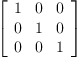(1)
Type: Matrix(NonNegativeInteger?)
fricas
CLDQ := CliffordAlgebra(3, Fraction(Integer), diagMat)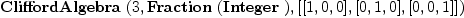(2)
Type: Type

CLDQ contains now the Clifford algebra constructor for the Clifford algebra CL(R^3,Q) corresponding to the diagonal quadratic form in the basis of generators ei=e(i)

fricas
basGen: List CLDQ :=[1, e(1), e(2), e(1)*e(2), e(3), e(1)*e(3), e(2)*e(3), e(1)*e(2)*e(3)]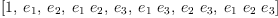(3)
Type: List(CliffordAlgebra?(3,Fraction(Integer),[[1,0,0],[0,1,0],[0,0,1]]))

And we can now compute within this basis

fricas
elem1: CLDQ :=2*e(1)+e(2)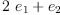(4)
Type: CliffordAlgebra?(3,Fraction(Integer),[[1,0,0],[0,1,0],[0,0,1]])
fricas
elem2: CLDQ :=1/2+e(2)+3*e(1)*e(3)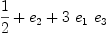(5)
Type: CliffordAlgebra?(3,Fraction(Integer),[[1,0,0],[0,1,0],[0,0,1]])
fricas
elem1*elem2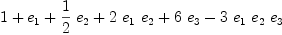(6)
Type: CliffordAlgebra?(3,Fraction(Integer),[[1,0,0],[0,1,0],[0,0,1]])

which is fine.

Lets now see what happens if we change the basis. We define new generators

fricas
f1:CLDQ := e(1)-e(2)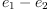(7)
Type: CliffordAlgebra?(3,Fraction(Integer),[[1,0,0],[0,1,0],[0,0,1]])
fricas
f2:CLDQ := e(2)-e(3)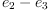(8)
Type: CliffordAlgebra?(3,Fraction(Integer),[[1,0,0],[0,1,0],[0,0,1]])
fricas
f3:CLDQ := e(1)+e(2)+e(3)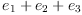(9)
Type: CliffordAlgebra?(3,Fraction(Integer),[[1,0,0],[0,1,0],[0,0,1]])
fricas
lstFGen: List CLDQ := [f1, f2, f3]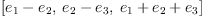(10)
Type: List(CliffordAlgebra?(3,Fraction(Integer),[[1,0,0],[0,1,0],[0,0,1]]))

and check what the new defining relations are

fricas
matrix [[y*x for x in lstFGen] for y in lstFGen]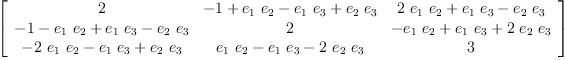(11)
Type: Matrix(CliffordAlgebra?(3,Fraction(Integer),[[1,0,0],[0,1,0],[0,0,1]]))

However, let us do the same calculations with another, symmetric but not diagonal quadratic form

fricas
offDiagMat:=matrix[[0,0,1],[0,1,0],[1,0,0]]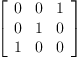(12)
Type: Matrix(NonNegativeInteger?)
fricas
CLODQ := CliffordAlgebra(3, Fraction(Integer), offDiagMat)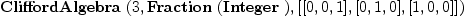(13)
Type: Type
fricas
basGenO: List CLODQ :=[1, e(1), e(2), e(1)*e(2), e(3), e(1)*e(3), e(2)*e(3), e(1)*e(2)*e(3)]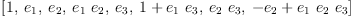(14)
Type: List(CliffordAlgebra?(3,Fraction(Integer),[[0,0,1],[0,1,0],[1,0,0]]))
fricas
basO: List CLODQ :=[e(1), e(2), e(3)]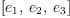(15)
Type: List(CliffordAlgebra?(3,Fraction(Integer),[[0,0,1],[0,1,0],[1,0,0]]))

and let us check the multiplication table on the basis and in general:

fricas
matrix [[y*x for x in basO] for y in basO]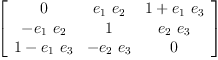(16)
Type: Matrix(CliffordAlgebra?(3,Fraction(Integer),[[0,0,1],[0,1,0],[1,0,0]]))
fricas
matrix [[y*x for x in basGenO] for y in basGenO]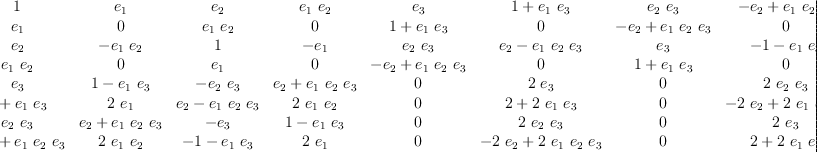(17)
Type: Matrix(CliffordAlgebra?(3,Fraction(Integer),[[0,0,1],[0,1,0],[1,0,0]]))

Note: Before Martin Baker fixed CliffordAlgebra? the results with off diagonal form were wrong.

 Subject:   Be Bold !! ( 14 subscribers )